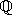## CGAL::Homogeneous<RingNumberType>

#include <CGAL/Homogeneous.h>

### Definition

A model for a Kernel using homogeneous coordinates to represent the geometric objects. In order for Homogeneous<RingNumberType> to model Euclidean geometry in E2 and/or E3, for some mathematical ring E (e.g., the integersor the rationals), the template parameter RingNumberType must model the mathematical ring E. That is, the ring operations on this number type must compute the mathematically correct results. If the number type provided as a model for RingNumberType is only an approximation of a ring (such as the built-in type double), then the geometry provided by the kernel is only an approximation of Euclidean geometry.

Kernel

### Types

 typedef Quotient FT; typedef RingNumberType RT;

### Implementation

This model of a kernel uses reference counting.